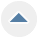# Math Fun: Multiplying By Nine

Category Education

For the early stages of learning math, this is pretty interesting.

The sum of any number multiplied by 9 will equal 9.

9x2=18
1+8=9

9x3=27
2+7=9

9x6=54
5+4=9

9x9+81
8+1=9

9x567=5103
5+1+0+3=9

9x1481=13,329
1+3+3+2+9=18, 1+8+9

and so on, and so on.

This doesn't really help with multiplication tables, but it's very cool.

By Sharon from Silver Spring MD

May 20, 20100 found this helpful

Hi Sharon,

It can be a great way to remember the 9 x table because if you notice that the answer starts with one number lower than what is being multiplied by nine ..... and then both numbers add up to nine.

For example:
9 x 7 = (one less than 7 is 6) and then adds up to nine. (6 + 3 = 9) answer is 63.

9 x 4 = (one less than 4 is 3) (3+6=9) answer is 36.

9x6 = (one less than 6 is 5) (5+4=9) answer is 54

etc.

I hope I have explained that ok :-)

May 21, 20100 found this helpful

Also if you work with numbers a lot and have tried to figure out if a difference is due to a transposition of numbers or something else, divide by 3. If the difference is evenly divided by 3 then it is probably transposition.

August 16, 20110 found this helpful

For the younger children, I make up a large poster board with the following:

9 x 1 = 9
9 x 2 - 18
9 x 3 = 27
9 x 4 = 36

Keep going and notice that while the first number in the answer gets larger, the second number gets smaller. I put the board up on the wall by the kitchen table and we read them off while eating breakfast. This worked for my children.

As they got older, I told them to memorize at least one equation in the middle and that would help bring back the rest in a test situation.
The memory board works for all the times tables. Helps to remember them when they are larger than life in front of you for a week at a time.